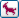Join the forum, it's quick and easy

Would you like to react to this message? Create an account in a few clicks or log in to continue.

# [分享]座標式標註 座標如果是負值 數字前自動加負號

+7
crihy
lcskc
junbafa74
jenniferaptg
qaz6605
edwei206
djh1006
11 posters##[分享]座標式標註 座標如果是負值 數字前自動加負號

[討論]請問座標式標註 座標如果是負值 請問要怎麼顯示呢?(詳圖)

`;DX.lsp;X軸座標_負號;載入後輸入DX執行(defun C:DX(/ LL OSP OCT XY CL PT1 TIMES DIMXY N1 NN1 P1 P2 PTN PTN1)  (graphscr)  (setvar "CMDECHO" 0);===新增圖層DIM===  (command "-LAYER" "P" "N" "0" "")  ;將圖層0設為不出圖  (setq LAY_CURRENT (getvar "CLAYER"))  ;取得目前圖層名稱  (command "-LAYER" "ON" LAY_CURRENT "T" LAY_CURRENT "U" LAY_CURRENT "")  ;將目前圖層打開並解鎖,解凍  (setq LL (tblsearch "LAYER" "DIM"))  ;偵測是否有DIM圖層<可設定成自己習慣圖層>  (if (= LL nil)(command "-LAYER" "N" "DIM" ""))  ;若無DIM層則新建DIM圖層<可設定成自己習慣圖層>  (command "-LAYER" "ON" "DIM" "T" "DIM" "U" "DIM" "C" "2" "DIM" "");打開DIM並解鎖,解凍<可設定成自己習慣圖層>;===  (setq    OSP (getvar "OSMODE")    OCT (getvar "CTAB")  ;確認目前在模型空間或配置空間    OCV (getvar "CVPORT")  ;當在模型空間時,值為2,在配置空間的圖紙空間時,值為1,在配置空間的視埠裡時,值為2    PT1 nil PT2 nil XY XY_record CL 1 TIMES 1  )  (if (= XY_record nil) (setq XY 1)(setq XY XY_record))  (while (= CL 1)  (while (= PT1 nil)    (if (= XY 1)(progn      (initget "U u")      (setq PT1 (getpoint "\n▍▍▍▍▍X軸標註點[Y軸標註(ENTER)  復原(U)  結束(ESC)]："))      (if (or (= PT1 "U")(= PT1 "u")) (command "undo" "1"))      (while (or (= PT1 "U")(= PT1 "u"))        (initget "U u")        (setq PT1 (getpoint "\n▍▍▍▍▍X軸標註點[Y軸標註(ENTER)  復原(U)  結束(ESC)]："))        (if (or (= PT1 "U")(= PT1 "u")) (command "undo" "1"))      )      (if (and (/= PT1 nil)(or (/= PT1 "u")(/= PT1 "U")))(progn          (setq XY_record XY DIMXY "X" N1 (car PT1) TIMES 1)      ))        (if (= PT1 nil)(progn            (setq XY 2 TIMES (1+ TIMES))            (if (= TIMES 5) (exit))        ))      ))  ;end if      (if (= XY 2)(progn          (initget "U u")          (setq PT1 (getpoint "\n▄▄▄▄▄Y軸標註點[X軸標註(ENTER)  復原(U)  結束(ESC)]："))          (if (or (= PT1 "U")(= PT1 "u")) (command "undo" "1"))          (while (or (= PT1 "U")(= PT1 "u"))           (initget "U u")            (setq PT1 (getpoint "\n▄▄▄▄▄Y軸標註點[X軸標註(ENTER)  復原(U)  結束(ESC)]："))            (if (or (= PT1 "U")(= PT1 "u")) (command "undo" "1"))          )          (if (and (/= PT1 nil)(or (/= PT1 "u")(/= PT1 "U")))(progn              (setq XY_record XY DIMXY "Y" N1 (cadr PT1) TIMES 1)          ))  ;end if          (if (= PT1 nil)(progn              (setq XY 1 TIMES (1+ TIMES))              (if (= TIMES 5) (exit))          )) ;end if      ))  ;end if    )    (while (and (/= PT1 nil)(or (/= PT1 "u")(/= PT1 "U")))      (command "UNDO" "BE")      (cond         ((< N1 0)   (setq NN1 "-<>"))        ((>= N1 0)   (setq NN1 ""))      )      (command "DIMORDINATE" PT1 DIMXY "T" NN1 pause)      (setvar "OSMODE" 0)      (if (= OCT "Model")(progn        (setq P1 (polar PT1 (/ (* 135 PI) 180) 10))        (setq P2 (polar PT1 (/ (* 315 PI) 180) 10))        (command "ZOOM" P1 P2)      ))      (setq PTN (ssget PT1))      (if (/= PTN nil)(progn          (setq PTN1 (cdr (assoc 0 (entget (ssname PTN 0)))))          (if (= PTN1 "DIMENSION")            (command "CHANGE" PT1 "" "P" "LA" "DIM" "C" "BYLAYER" "LT" " BYLAYER" "")          )      ))      (if (= OCT "Model")(command "ZOOM" "P"))      (setvar "OSMODE" OSP)      (setq PT1 nil)      (command "UNDO" "E")    )  ;end while  )  ;end while  (princ))`

Tiger&蘋果爸 寫到:謝謝熱心程式分享~< 積分 +1>
[公告]關於團隊成員的回文及貢獻

____________________________________________________________________________________djh1006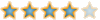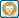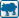##回復： [分享]座標式標註 座標如果是負值 數字前自動加負號edwei206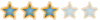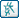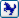##回復： [分享]座標式標註 座標如果是負值 數字前自動加負號qaz6605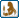##回復： [分享]座標式標註 座標如果是負值 數字前自動加負號jenniferaptg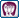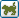##回復： [分享]座標式標註 座標如果是負值 數字前自動加負號

LISP太複雜，希望你能找到解答，感謝分享～junbafa74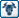##回復： [分享]座標式標註 座標如果是負值 數字前自動加負號lcskc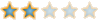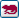##回復： [分享]座標式標註 座標如果是負值 數字前自動加負號crihy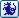##回復： [分享]座標式標註 座標如果是負值 數字前自動加負號homebrown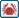##回復： [分享]座標式標註 座標如果是負值 數字前自動加負號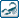##回復： [分享]座標式標註 座標如果是負值 數字前自動加負號Tiger&蘋果爸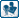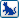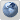##回復： [分享]座標式標註 座標如果是負值 數字前自動加負號nashc